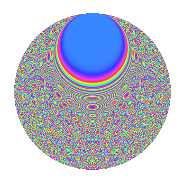# Properties

 Label 2541.2.biLevel 2541 Weight 2 Character orbit bi Rep. character $$\chi_{2541}(40,\cdot)$$ Character field $$\Q(\zeta_{30})$$ Dimension 1152 Sturm bound 704

# Related objects

## Defining parameters

 Level: $$N$$ = $$2541 = 3 \cdot 7 \cdot 11^{2}$$ Weight: $$k$$ = $$2$$ Character orbit: $$[\chi]$$ = 2541.bi (of order $$30$$ and degree $$8$$) Character conductor: $$\operatorname{cond}(\chi)$$ = $$77$$ Character field: $$\Q(\zeta_{30})$$ Sturm bound: $$704$$

## Dimensions

The following table gives the dimensions of various subspaces of $$M_{2}(2541, [\chi])$$.

Total New Old
Modular forms 3008 1152 1856
Cusp forms 2624 1152 1472
Eisenstein series 384 0 384

## Trace form

 $$1152q - 148q^{4} - 12q^{5} + 10q^{7} + 40q^{8} - 144q^{9} + O(q^{10})$$ $$1152q - 148q^{4} - 12q^{5} + 10q^{7} + 40q^{8} - 144q^{9} - 28q^{14} - 12q^{15} + 112q^{16} + 60q^{17} + 10q^{18} + 24q^{23} + 90q^{24} - 140q^{25} - 24q^{26} - 30q^{28} - 40q^{29} + 18q^{31} + 80q^{35} - 296q^{36} + 12q^{37} + 24q^{38} + 90q^{40} - 22q^{42} - 12q^{45} - 70q^{46} + 36q^{47} + 98q^{49} + 20q^{51} - 8q^{53} + 232q^{56} + 48q^{58} - 96q^{59} - 12q^{60} - 30q^{61} + 10q^{63} + 360q^{64} + 56q^{67} - 180q^{68} - 154q^{70} + 64q^{71} - 10q^{72} - 90q^{73} - 40q^{74} + 48q^{75} + 168q^{78} - 50q^{79} - 276q^{80} + 144q^{81} - 192q^{82} + 60q^{84} + 20q^{85} - 138q^{86} - 48q^{89} + 16q^{91} + 204q^{92} - 8q^{93} - 10q^{95} + O(q^{100})$$

## Decomposition of $$S_{2}^{\mathrm{new}}(2541, [\chi])$$ into newform subspaces

The newforms in this space have not yet been added to the LMFDB.

## Decomposition of $$S_{2}^{\mathrm{old}}(2541, [\chi])$$ into lower level spaces

$$S_{2}^{\mathrm{old}}(2541, [\chi]) \cong$$ $$S_{2}^{\mathrm{new}}(77, [\chi])$$$$^{\oplus 4}$$$$\oplus$$$$S_{2}^{\mathrm{new}}(231, [\chi])$$$$^{\oplus 2}$$$$\oplus$$$$S_{2}^{\mathrm{new}}(847, [\chi])$$$$^{\oplus 2}$$

## Hecke Characteristic Polynomials

There are no characteristic polynomials of Hecke operators in the database